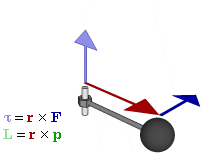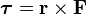# Torque facts for kids

Kids Encyclopedia FactsRelationship between force, torque, and momentum vectors in a rotating system

In physics, torque is the tendency of a force to turn or twist. If a force is used to begin to spin an object, or to stop an object from spinning, a torque is made.

The force applied to a lever, multiplied by the distance from the lever's fulcrum, multiplied again by the sine of the angle created, is described as torque. This is also known as "r cross f," or "force times fulcrum distance times sine theta."

## Fulcrum

Fulcrum is the axis of rotation or point of support on which a lever turns in raising or moving something.

## Equation

The equation for torque is:$\boldsymbol \tau = \mathbf{r}\times \mathbf{F}\,\!$

where F is the force vector and r is the vector from the axis of rotation to the point where the force is acting.

The units of torque are force multiplied by distance. The SI unit of torque is the newton-metre. The most common English unit is the foot-pound.Torque Facts for Kids. Kiddle Encyclopedia.